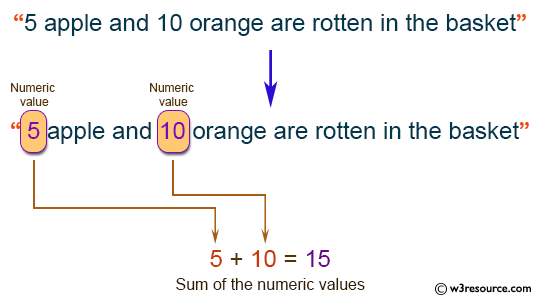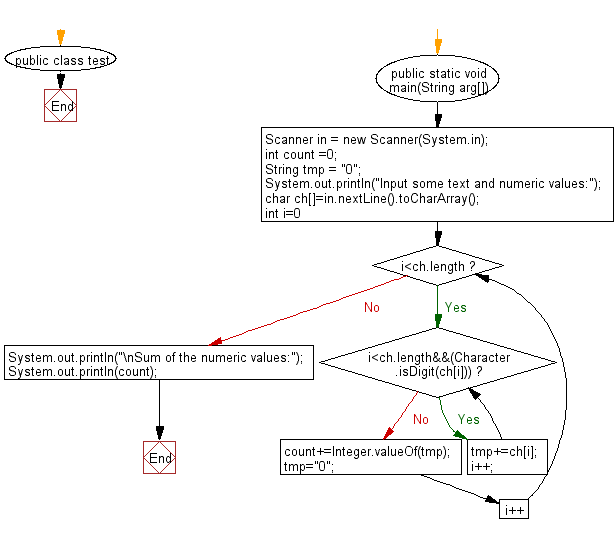# Java Exercises: Sum of all numerical values embedded in a sentence

## Java Basic: Exercise-236 with Solution

Write a Java program to sum of all numerical values (positive integers) embedded in a sentence.

Input:
Sentences with positive integers are given over multiple lines. Each line is a character string containing one-byte alphanumeric characters, symbols, spaces, or an empty line. However the input is 80 characters or less per line and the sum is 10,000 or less.

Pictorial Presentation:Sample Solution:

Java Code:

`````` import java.util.Scanner;
public class Main
{
public static void main(String arg[])
{
Scanner in = new Scanner(System.in);
int count =0;
String tmp = "0";
System.out.println("Input some text and numeric values:");
char ch[]=in.nextLine().toCharArray();
for(int i=0; i<ch.length; i++)
{
while(i<ch.length&&(Character.isDigit(ch[i])))
{
tmp+=ch[i];
i++;
}
count+=Integer.valueOf(tmp);
tmp="0";
}
System.out.println("\nSum of the numeric values:");
System.out.println(count);
}
}
```
```

Sample Output:

```Input some text and numeric values:
5 apple and 10 orange are rotten in the basket

Sum of the numeric values:
15
```

Flowchart:Java Code Editor:

What is the difficulty level of this exercise?

﻿

## Java: Tips of the Day

Directory Content:

Java allows you to get the names of all subdirectories and files in a folder as an array, which can then be sequentially viewed.

```import java.io.*;

public class ListContents {
public static void main(String[] args) {
File file = new File("//home//user//Documents/");
String[] files = file.list();

System.out.println("Listing contents of " + file.getPath());
for(int i=0 ; i < files.length ; i++)
{
System.out.println(files[i]);
}
}
}
```